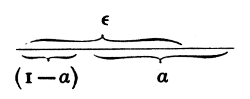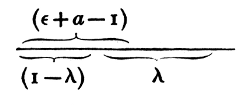#Pillow-Problems: Problem #25

Math Lair Home > Source Material > Pillow-Problems > Problem #25

## Problem:

25.

If 'ε', 'α', 'λ' represent proper fractions; and if, in a certain hospital, 'ε' of the patients have lost an eye, 'α' an arm, and 'λ' a leg: what is the least possible number who have lost all three?

[7/2/76

25.

ε + α + λ − 2.

## Solution:

25.

Let ‘E’ mean ‘having lost an eye’, ‘A’ ‘having lost an arm’, and ‘L’ ‘having lost a leg’.

Then the state of things which gives the least possible number of those who, being E and A, are also L, may evidently be found by arranging the patients in a row, so that the EA-class may begin from one end of the row, and the L-class from the other end, and counting the portion where they overlap; and, the smaller the EA-class, the smaller will be this common portion: hence we must make the EA-class the minimum.This may be done by re-arranging the patients, so that the E-class may begin from one end of the row, and the A-class from the other: and the least possible number for the EA-class is the common portion, i.e. (ε − 1 − α), i.e. (ε + α − 1).Then, as already shown, the least possible number for the EAL-class is the common portion, i.e. (ε + α − 1 − 1 − λ), i.e. (ε + α + λ − 2).

Q.E.F.